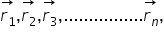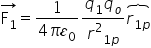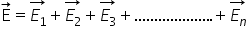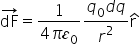# Electric Field due to charges

Electric field due to a point charge:

As shown in fig. consider a point charge q placed at the origin o. we wish to determine its electric field at a point P at distance r form it. For this, imagine a test charge q0 placed at point p. according to the coulomb’s law, the force on charge q0 is

this means that at all points on the spherical surface drawn around the point charge, the magnitude is same and does not depend on the direction of r. such a field is called spherically symmetric or radial field. i.e. a field which looks the same in all directions when seen from the point charge.

Electric field due to a system of point charges:

As shown in fig. consider N point charges q1, q2, q3, ………..qn, place in vacuum at points whose position n vectors w.r.t. origin o arerespectively according to the coulomb’s law the force on charge q0 due to charge q1 isThe electric field at pint p due to charge q1 is

According to the principle of superposition, the electric field at point P to a system of N charges isin terms of position vectors,

Continuous Charge Distribution:

In practice, we deal with charges much greater in magnitude than the charge on an electron, so we can ignore the quantum nature of charges and imagine that the charge is spread in a region in a continuous manner. Such a charge distribution is known as continuous charge distribution.

Force on O point charge qo due to continuous charge distribution

As shown in fig. consider a point charge q0 lying near a region of continuous charge distribution. This continuous charge distribution can be imagined to consist of a large number of small charges dq. According to the coulomb’s law, the force on charge q0 due to small charge dq isBy the principle of superposition , the total force on charge q0 will be vector sum of the force exerted by all such small charges and is given by

Related Keywords